# Witt decomposition

(diff) ← Older revision | Latest revision (diff) | Newer revision → (diff)

of a vector space

A decomposition of the space into a direct sum of three subspaces having certain properties. More exactly, letbe a vector space over a fieldwith characteristic different from 2, provided with a metric structure by means of a symmetric or skew-symmetric bilinear form. The direct decompositionis said to be a Witt decomposition ofifand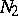are totally isotropic, whileis anisotropic and is orthogonal to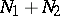with respect to. The Witt decomposition plays an important role in the study of the structure of the formand in problems of classification of bilinear forms.

Letbe a non-degenerate bilinear form and letbe finite-dimensional. Then any maximal totally isotropic subspace inmay be included in a Witt decomposition ofasor. For any Witt decomposition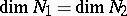, and for any basis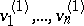in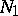, there exists a basis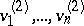insuch that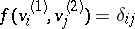(are the Kronecker symbols). For any two Witt decompositions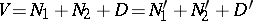the condition,is necessary and sufficient for the existence of a metric automorphismofsuch that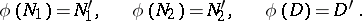A non-degenerate symmetric or skew-symmetric bilinear formonis said to be neutral ifis finite-dimensional and has a Witt decomposition with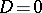. In this case the symmetric form is said to be a hyperbolic form, whileis said to be a hyperbolic space. An orthogonal direct sum of neutral forms is neutral. The matrix of a neutral form (in the basisof the space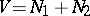described above) looks likewhereis the identity matrix of order, while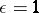for a symmetric form and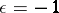for a skew-symmetric form. Two neutral forms are isometric if and only if they have the same rank. The class of neutral symmetric bilinear forms is the zero (i.e. the neutral element for addition) in the Witt ring of the field. Neutral forms and only such forms have Witt index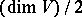. A skew-symmetric form on a finite-dimensional space is neutral.

Ifis a non-degenerate symmetric bilinear form on a finite-dimensional spaceand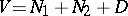is a Witt decomposition in whichis equal to the Witt index of, the restriction oftois a definite, or anisotropic, bilinear form, i.e. is such thatfor all non-zero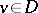. This form is independent (apart from an isometry) of the choice of the Witt decomposition of. In the set of definite bilinear forms it is possible to introduce an addition, converting it into an Abelian group — the Witt group of(cf. Witt ring).

Let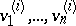be bases in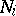,, such that; the union of these bases with an arbitrary basis inyields a basis inin which the matrix oflooks like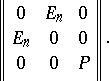For symmetric bilinear forms there exists an orthogonal basis in, i.e. a basis in which the matrix of the form is diagonal. If the fieldis algebraically closed, there even exists an orthonormal basis (a basis in which the matrix of the form is the identity), and for this reason two non-degenerate symmetric bilinear forms of finite rank overare isometric if and only if they have the same rank. In the general case the classification of such forms substantially depends on the arithmetical properties of the field.

The study and classification of degenerate symmetric and skew-symmetric forms can be reduced to the study of non-degenerate forms (the restriction of the form to a subspace which is complementary to the kernel of the form).

All what has been said above permits a generalization to the case of-Hermitian forms over a skew-field with property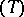(cf. Witt theorem), and also to the case of symmetric bilinear forms associated with quadratic forms, without restrictions on the characteristic of the field.

How to Cite This Entry:
Witt decomposition. Encyclopedia of Mathematics. URL: http://encyclopediaofmath.org/index.php?title=Witt_decomposition&oldid=17145
This article was adapted from an original article by V.L. Popov (originator), which appeared in Encyclopedia of Mathematics - ISBN 1402006098. See original article